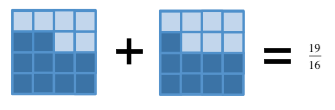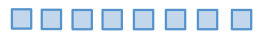### Home > CC2 > Chapter Ch2 > Lesson 2.3.1 > Problem2-117

2-117.Copy each expression and then simplify it without using a calculator. Be sure to show all steps. Homework Help ✎

1. $7\frac{5}{8}+2\frac{9}{16}$

This sum can be represented with a diagram:

Find a common denominator to add the two fractions.2. $2\frac{7}{8}+6\frac{1}{6}$

See part (a) for strategy.

3. $8-6\frac{2}{5}$

Draw $8$ squares on your paper like this:

Cross off $6\frac{2}{5}$. What is left?$1\frac{3}{5}$